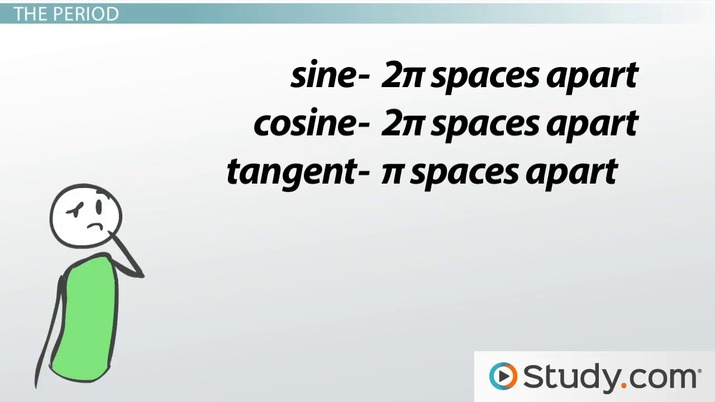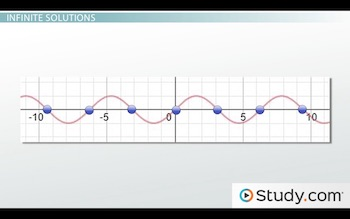# Solving Trigonometric Equations with Infinite Solutions

Lesson Transcript
Instructor: Yuanxin (Amy) Yang Alcocer

Amy has a master's degree in secondary education and has been teaching math for over 9 years. Amy has worked with students at all levels from those with special needs to those that are gifted.

In trigonometry, equations have an infinite number of solutions. In this lesson, review the period, explore sine, cosine, and tangent, and work through examples to understand the different ways that trigonometric equations can be solved. Updated: 10/27/2021

## Trigonometric Equations

In this video lesson we talk about trigonometric equations. What are they? Remember your basic trig functions of sine, cosine, and tangent? Well, your trigonometric equations are simply equations that include trig functions. For example, the equation sin x = 1 is an example of a trigonometric equation. Do you see the sine function in this equation?

You will know that you are looking and working with a trigonometric equation if you see any one of the trig functions in the equation or any combination thereof. We have six of them. They are sine, cosine, tangent and their reciprocals of cosecant, secant, and cotangent. One more thing: trigonometric equations will also have the variable as part of the argument of the trig function. Like, in our example we had sine of x where x is the argument of the sine function.

To help us solve these types of problems, we can use the inverse trig function buttons on our calculator, a graphing calculator, or we can use the unit circle, our special circle of radius of 1, that has solutions to common angles for the cosine and sine functions. Keep watching and you will find out which one of these methods is the best.An error occurred trying to load this video.

Try refreshing the page, or contact customer support.

Coming up next: Finding the Sinusoidal Function

### You're on a roll. Keep up the good work!

Replay
Your next lesson will play in 10 seconds
• 0:01 Trigonometric Equations
• 1:09 Infinite Solutions
• 2:41 The Period
• 3:35 Example
• 5:27 Lesson Summary
Save Save

Want to watch this again later?

Timeline
Autoplay
Autoplay
Speed Speed

## Infinite Solutions

There is one interesting characteristic that trigonometric equations have. And that is that they have an infinite number of solutions. You might think this sounds weird, especially since your calculator only returns one answer. Let's look at the graph of the sine function and you will see something interesting:Do you see the waves? Yes, our sine function graphs out as waves. Do you see that these waves keep crossing our x-axis? Well, every time our graph crosses the x-axis, when y = 0, it gives us another solution. This graph keeps on going forever. What you get when you calculate inverse trig functions in your calculator is the answer closest to the origin. So, if I wanted to solve sin x = 0, I would take the inverse sine of 0. I get an answer of 0 on my calculator, but in reality, sin x = 0 has an infinite number of solutions. As you can see on the graph, I get 0 for sin x every 2pi spaces. So my answers are actually x = 0 + 2*pi*n where n stands for the number of extra solutions that we have. N increases by 1 each time. We get x = 0 + 2*pi*0 = 0 for our first solution. The next solution is x = 0 + 2*pi*1 = 2pi. The next one is x = 0 + 2*pi*2 = 4pi. And so on and so forth.

## The Period

This spacing is the period. It is different depending on the trig function we are using. The sine and cosine functions both have a period of 2pi. This means that your answers are 2pi spaces apart. The tangent function, on the other hand, has a period of pi. This means that these answers are pi spaces apart. These are the standard periods of our trig functions. These periods can be changed if our trigonometric equation is more complex. But, we won't discuss that in this video lesson.

So for this video lesson, focus on learning the standard period of the sine, cosine, and tangent functions. Just remember that sine and cosine share a period of 2pi while tangent (which doesn't sound like the other two) has a period of just pi.

## Example

Let's look at how we use this information now.

Solve 2 cos x - 1 = 0.

To unlock this lesson you must be a Study.com Member.

### Register to view this lesson

Are you a student or a teacher?